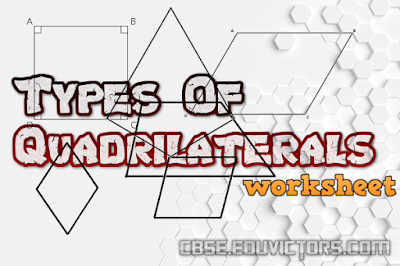## Mathematics - Types of Quadrilaterals

(Worksheet)

Fill in the blanks.

1. A quadrilateral with all angles 90° is either a _______ or a _________.

2. In a parallelogram opposite angles are ______.

3. In a parallelogram adjacent angles are ______.

4. A quadrilateral with all sides equal is either a _______ or a ________.

5. A square is a rectangle in which ______________________________.

6. A parallelogram has both pairs of opposite sides ________ and  _________.

7. A quadrilateral having exactly one pair of parallel sides is called a _________.

8. A quadrilateral in which the diagonals bisect each other at right angles is either a ________ or a __________.

9. Every rhombus is a _______ as well as ________.

10. A quadrilateral in which diagonals bisect each other is either a ______, _______, _______.

11. Every _______ is a rhombus.

12. Every _______ is a rectangle.

13. The sum of all angles of a quadrilateral is _______.

14. ________ is a regular quadrilateral.

15. Diagonals of a rectangle are————.

1. rectangle, square
2. equal
3. supplementary
4. rhombus, square
6. equal, parallel
7. trapezium
8. rhombus, square
9. parallelogram, kite
10. parallelogram, rhombus, square, rectangle.
11. square
12. square
13. 360°
14. Square
15. equal.